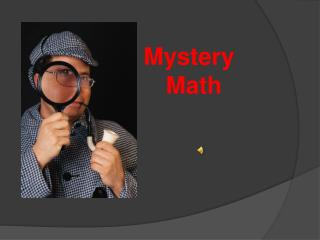# Mystery Math - PowerPoint PPT PresentationDownload PresentationMystery Math

Mystery MathDownload Presentation## Mystery Math

- - - - - - - - - - - - - - - - - - - - - - - - - - - E N D - - - - - - - - - - - - - - - - - - - - - - - - - - -
##### Presentation Transcript

1. Mystery Math

2. readerEreader

3. Lynn Angelovich, M.Ed Math Terms for 4th Grade

4. Why should we study the meaning of math words, anyway?

5. to keep busy, it doesn’t matter, I will guess anyway… I don’t need to learn math and who cares? to understand what is being asked math concepts are used by most people, every day

6. Shop Wisely

7. cooking design games

8. The top 15 highest earning degrees have one thing in common… math skills. -National Association of College Employers

9. sum

10. the result of adding numbers 4 + 5 = 9

11. Sum or Not? 4 x 5 = 20 100 + 256 = 356 4 + 2 = 6 297 – 97 = 197 18 – 3 = 15 2975 + 25 = 3000 20 x 3 = 60 892 – 92 = 800

12. difference

13. the result of subtracting

14. difference? yes or no 1000 – 900 = 100 20,057 – 1002 = 19,055 12 x 6 = 72 822 – 122 = 700 582 + 100 = 682

15. product

16. the result of multiplication

17. product? 25 x 3 = 75 1000 – 50 = 950 80 x 3 = 240 8 x 7 = 56 333 + 57 = 390 100/2 = 50 800 + 750 = 1550 10 x 5 = 50

18. value

19. quantity represented by numbers 4 + x = 6

20. fact family

21. a set of related equations that use the same numbers division multiplication

22. fact family ? mathematicsdictionary.com primarysourcesresourceprimary.co.uk

23. Presentation created with Microsoft 2007 Clip Art